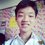# An Interesting Type of Inequalities

(Vasile Cirtoaje) If $$a,b,c\in \left[\frac{1}{\sqrt 2}, \sqrt 2\right],$$ show that $\frac{3}{a+2b}+\frac{3}{b+2c}+\frac{3}{c+2a}\geqslant \frac{2}{a+b}+\frac{2}{b+c}+\frac{2}{c+a}$ holds.

$\color{#D61F06}{\textbf{Solution:}}$ Write the inequality as $\sum_{cyc} \left(\frac{3}{a+2b}-\frac{2}{a+b}+\frac{1}{6a}-\frac{1}{6b}\right)\geqslant 0$ or $\sum_{cyc} \frac{(a-b)^2(2a-b)}{6ab(a+2b)(a+b)}\geqslant 0.$ Since $2a-b \geqslant 2 \cdot \frac{1}{\sqrt 2}-\sqrt 2=0,$ the inequality holds obviously. $\quad \blacksquare$

However, you may wonder, how in the world do we know that adding $\frac{1}{6a}$ and subtracting $\frac{1}{6b}$ helps? Well, let's go back to our initial inequality, $\frac{3}{a+2b}+\frac{3}{b+2c}+\frac{3}{c+2a}\geqslant \frac{2}{a+b}+\frac{2}{b+c}+\frac{2}{c+a}$ the motivation behind the solution is that if we can somehow turn it to a cyclic sum of non-negative terms, (which contain some squares in them) then we're done! So, intuitively, let's try to just move the RHS terms to the LHS, and focus on just two variables, $a$ and $b$, (since the sum is cyclic) $\sum_{cyc} \left(\frac{3}{a+2b}-\frac{2}{a+b}\right)\geqslant 0$ or $\sum_{cyc} \frac{b-a}{(5a+b)(4a+b)}\geqslant 0.$ No good here, as we cannot guarantee each term of the cyclic sum to be non-negative.

Now, why don't we manipulate the sum a little? Let's add $\frac{k}{a}-\frac{k}{b}$ cyclically to the sum (which is just $0$) and then if we can find a suitable $k$, then we're done right?

So, we start by writing down, $\sum_{cyc} \left(\frac{3}{a+2b}-\frac{2}{a+b}+\frac{k}{a}-\frac{k}{b}\right)\geqslant 0$ or $\sum_{cyc} \frac{(a-b)(ab-k(a+2b)(a+b))}{ab(a+2b)(a+b)}\geqslant 0.$ Here, we can see that there's a $(a-b)$ term which is not always non-negative, but the square of it is non-negative by the trivially inequality. Thus, if we can have an extra factor of $(a-b)$, that may help us! Moreover, since $ab-k(a+2b)(a+b)$ is a polynomial in $a,b$, if we want to have a factor of $(a-b)$, we can treat it as a polynomial in $a$ and it is zero when $a=b$. Setting $a=b$, gives $a^2(1-6k)=0$, since $a\ne 0$, we can choose $k=\frac{1}{6}$.

When $k=\frac{1}{6}$, the inequality becomes $\sum_{cyc} \frac{(a-b)^2(2a-b)}{6ab(a+2b)(a+b)}\geqslant 0.$

Using this method, we can also prove some other similar inequalities:

1. Prove that if $a,b,c \in \left[\frac{1}{\sqrt 3}, \sqrt 3\right]$, then $\frac{5}{2a+3b}+\frac{5}{2b+3c}+\frac{5}{2c+3a}\leqslant \frac{3}{a+2b}+\frac{3}{b+2c}+\frac{3}{c+2a}.$

2. Prove that if $a,b,c \in \left[\frac{1}{2\sqrt 5}, 2\sqrt 5\right]$, then $\frac{6}{5a+b}+\frac{6}{5b+c}+\frac{6}{5c+a}\geqslant \frac{5}{4a+b}+\frac{5}{4b+c}+\frac{5}{4c+a}.$

3. Prove that if $a,b,c \in \left[\frac{1}{\sqrt {15}}, \frac{\sqrt {15}}{2}\right]$, then $\frac{7}{5a+2b}+\frac{7}{5b+2c}+\frac{7}{5c+2a}\leqslant \frac{4}{3a+b}+\frac{4}{3b+c}+\frac{4}{3c+a}.$Note by ChengYiin Ong
4 weeks, 1 day ago

This discussion board is a place to discuss our Daily Challenges and the math and science related to those challenges. Explanations are more than just a solution — they should explain the steps and thinking strategies that you used to obtain the solution. Comments should further the discussion of math and science.

When posting on Brilliant:

• Use the emojis to react to an explanation, whether you're congratulating a job well done , or just really confused .
• Ask specific questions about the challenge or the steps in somebody's explanation. Well-posed questions can add a lot to the discussion, but posting "I don't understand!" doesn't help anyone.
• Try to contribute something new to the discussion, whether it is an extension, generalization or other idea related to the challenge.
• Stay on topic — we're all here to learn more about math and science, not to hear about your favorite get-rich-quick scheme or current world events.

MarkdownAppears as
*italics* or _italics_ italics
**bold** or __bold__ bold
- bulleted- list
• bulleted
• list
1. numbered2. list
1. numbered
2. list
Note: you must add a full line of space before and after lists for them to show up correctly
paragraph 1paragraph 2

paragraph 1

paragraph 2

[example link](https://brilliant.org)example link
> This is a quote
This is a quote
    # I indented these lines
# 4 spaces, and now they show
# up as a code block.

print "hello world"
# I indented these lines
# 4 spaces, and now they show
# up as a code block.

print "hello world"
MathAppears as
Remember to wrap math in $$ ... $$ or $ ... $ to ensure proper formatting.
2 \times 3 $2 \times 3$
2^{34} $2^{34}$
a_{i-1} $a_{i-1}$
\frac{2}{3} $\frac{2}{3}$
\sqrt{2} $\sqrt{2}$
\sum_{i=1}^3 $\sum_{i=1}^3$
\sin \theta $\sin \theta$
\boxed{123} $\boxed{123}$

## Comments

There are no comments in this discussion.

×

Problem Loading...

Note Loading...

Set Loading...